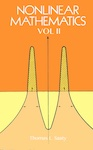# Books

##### Non Linear Mathematics Vol. IIAuthor(s)
Thomas Saaty
Publisher(s): RWS Publications
Language: English, American
Pages: 480
ISBN: 978-1-888603-39-2

Abstract: Nonlinear equations have existed for hundreds of years; their systematic study, however, is a relatively recent phenomenon. This volume, together with its companion', Nonlinear Matliematics Vol. I, provides exceptionally comprehensive coverage of this recently formed area of study. It encompasses both older and more recent developments in the field of equations, with particular emphasis on nonlinear equations because, as Professor Saaty maintains, that is what is needed today. Together the two volumes cover all the major types of classical equations (except partial differential equations, which require a separate volume). This volume includes material on seven types: operator equations, functional equations, difference equations, delay-differential equations, integral equations, integro-differential equations and stochastic differential equations. Special emphasis is placed on linear and nonlinear equations in function spaces and On general methods of solving different types of such equations. Above all, this book is practical. It reviews the variety of existing types of equations and provides methods for their solution. It is meant to help the reader acquire new methods for formulating problems. Its clear organization and copious references make it suitable for graduate students as well as scientists, technologists and mathematicians.

Subject(s): Non-linear mathematics